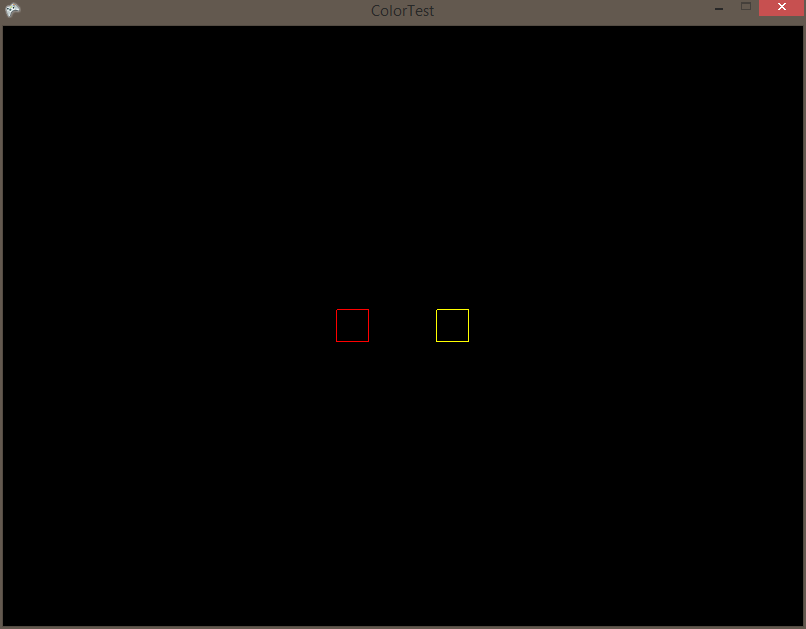# FlatRedBall.Math.Geometry.AxisAlignedRectangle.Color

## Introduction

The AxisAlignedRectangle object supports changing its Color property by assigning a Color value.

## Example

The following example requires that LeftRectangle and RightRectangle are valid Rectangles. For this example the rectangles were created in Glue. The following code can be used to modify the colors of the Rectangles: